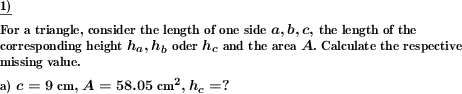Custom math worksheets at your fingertips# Details for problem "Triangle missing value area, side or height"

Quickname: 3335

Junior High School, Middle School, High School.

## Summary

One of the values area, length of a side or corresponding height of a triangle are given. Determine the missing value.

## Example## Description

Use this problem type on worksheets to calculate the area, the missing side, or height in a triangle when all other values are given.

This problem is about the formula for a triangle's area according to the formula A=c*hc/2. Two of the values involved are given, the third one is to be determined.

Two of these values are given for each problem: The length of the side, the length of the corresponding height, and the area. Which of these are given can be configured. The third value has to be calculated. This kind of worksheet can be used as a small or whole group activity or independent/ homework practice for mastery of finding the perimeter of a triangle using sides. Practice this worksheet in variation to boost the objectives given below.

1. Recall the multiplication and division of decimal numbers.
2. The formula to find the area of a triangle is revised.
3. Understand the use of measurement units.
4. Buildup concept of deriving required value from a given equation.
5. Develop algebraic skills in geometry.

The complexity level can be personalized to use these worksheets from grade 7 and grade 8.

• The number of problems is selectable from 1 to 10.
• As a small difficulty, useless values such as additional side length or height specifications can be scattered in.
• The correct specifications must then be selected for the calculation.

The answer key will include the solution of missing value.

Download free printable worksheets for this math problem here. The worksheet contains the problems only, the solution sheet includes the answers. Just click on the respective link.

•Worksheet 1Solution sheet with answers
•Worksheet 2Solution sheet with answers
•Worksheet 3Solution sheet with answers

If you can not see the solution sheets for download, they may be filtered out by an ad blocker that you may have installed. If this is the case, please allow ads for this page and reload the page. The solution sheets will then reappear.

• Do these sample worksheets do not really fit?
• Do you need more math worksheets, with a different level of difficulty?
• Would you like to combine different problems on a worksheet and adjust them to your needs?
• As a teacher, you can put together your own worksheets using the automatically generated math problems provided.
With a free initial credit, you can start creating your own math worksheets in a few minutes.

You can try it for free! Register here, to create custom worksheets now!

## Customization options for this problem

Parameter
Possible values
Number of problems
1, 2, 3, 4, 5, 6, 7, 8, 9, 10
Given values
any two, side and height, height and area, side and area